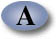Unified Engineering Spring Term 2004

Problem P3. (Propulsion) (LO A & B)

An incompressible fluid flows steadily through a two-dimensional infinite row of fixed airfoils (e.g. a stator blade row). The blade row is contained in a constant area annulus, as shown on the right side of the figure below. The spacing between the airfoils is s. Assume that the velocities and pressures Va, Vb, pa, pb, are constant at stations (a) and (b), and that the flow angles are given by ba and bb.a) Does the magnitude of the flow velocity increase/stay the same/decrease across the stator and why?

b) Using the control volume shown above (the upper and lower surfaces are streamlines), apply conservation of mass and momentum to determine the forces Rx and Ry that must be applied to the fluid (these are equal and opposite to the forces needed to keep each vane in place).